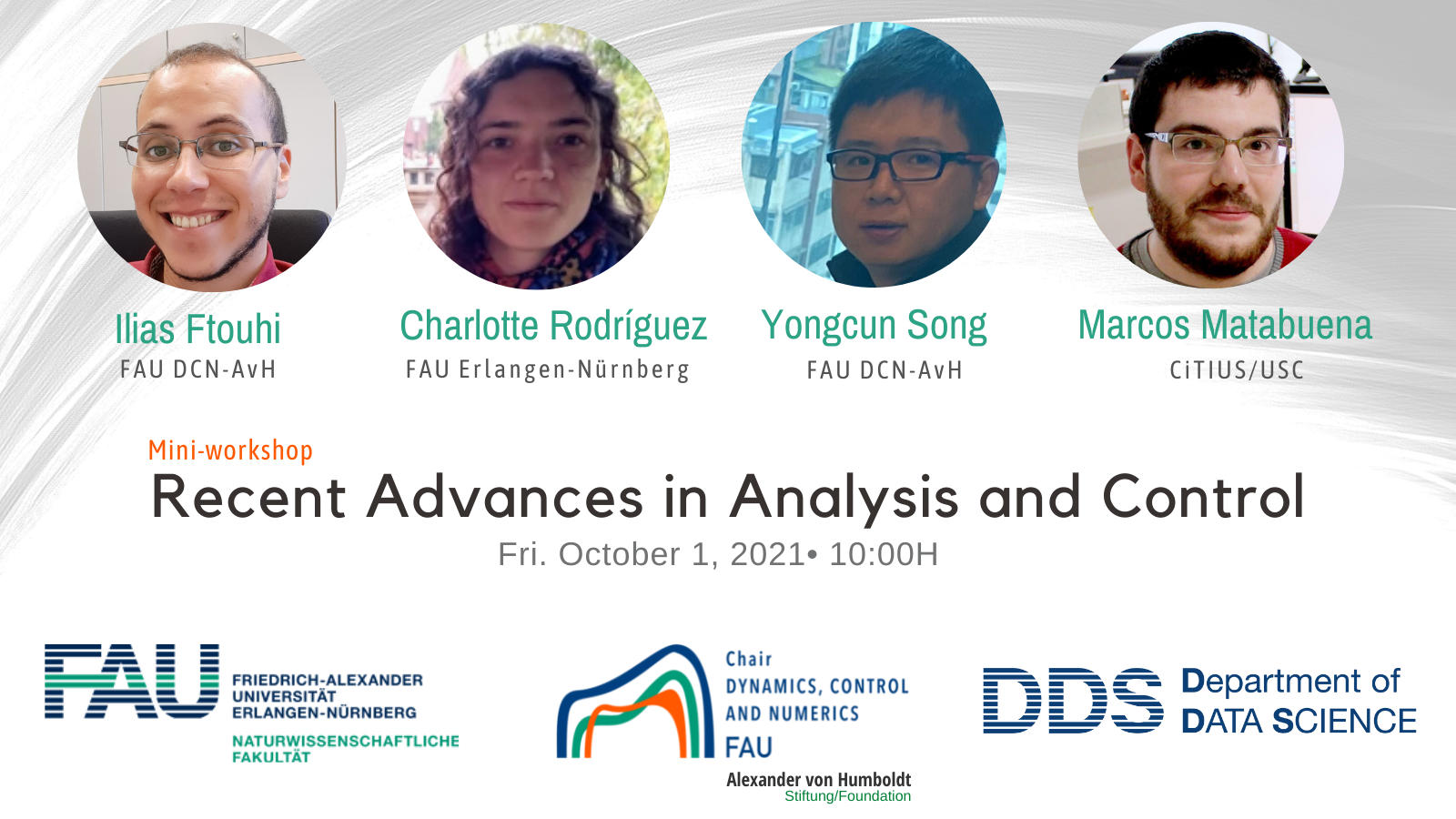# Mini-workshop: Recent Advances in Analysis and Control

Next Friday October 1, the FAU DCN-AvH, Chair for Dynamics, Control and Numerics – Alexander von Humboldt Professorship at FAU Erlangen-Nürnberg will host a mini-workshop:

10:00H – 10:30H
Ilias Ftouhi FAU DCN-AvH | FAU Erlangen-Nürnberg
“Blaschke-Santaló diagrams in spectral geometry”

Abstract. Spectral theory has been interesting various communities in the last century and still is. We are particularly interested in the spectrum of the Laplace operator with Dirichlet boundary conditions on partialOmega where Omegasubsetmathbb{R}^d, and more precisely its first eigenvalue also known as the fundamental frequency (lambda_1(Omega)). Unfortunately, for almost all given sets Omega, there is no explicit formula for lambda_1(Omega). This motivates to look for estimates via other functionals, which are much easier to compute (for example: Perimeter P(Omega) and measure |Omega|). First, we give a brief introduction on shape optimization and spectral theory, then we introduce the following set of points, which can be called the Blaschke-Santalo diagram of the triplet (lambda_1,P,|cdot|): mathcal{C}_{mathcal{F}_{ad}}:=big{ big(P(Omega),lambda_1(Omega)big) | Omega in mathcal{F}_{ad} text{and} |Omega|=1 big}, where mathcal{F}_{ad} is a given class of subsets of mathbb{R}^d. Notice that the characterization of such diagram is equivalent to finding all the possible scale-invariant inequalities between the three involved quantities (P, lambda_1 and |.| in our case). We are able to completely describe the diagram for open sets in mathbb{R}^d. It shows that there is no other inequality than the Faber-Krahn and the isoperimetric ones. This motivates us to investigate what happens for other classes of sets like the simply-connected and convex ones. We will give an advanced description of the Blaschke-Santalo diagram for convex sets in the plane. This work is in collaboration with Jimmy Lamboley.

10:30H – 11:00H
Charlotte RodríguezFAU Erlangen-Nürnberg
“Control and stabilization of geometrically exact beams”

Abstract. We will present well-posedness, boundary feedback stabilization and nodal profile control problems involving freely vibrating beams that may undergo motions of large magnitude (reference line’s displacement, cross sections’ rotations). These are so-called “geometrically exact beams” which are especially needed in modern highly flexible light-weight structures where one cannot neglect these large motions. The generally encountered mathematical model has a “wave-like” form (second-order in time and space, quasilinear, 6 governing equations) and describes the deflections of the beam. Here, we take advantage of another perspective where the beam is rather described in terms of velocities and strains, all moreover expressed in a moving coordinate system attached to the beam. Then, the model is of first-order, hyperbolic, semilinear and with 12 governing equations, but remains related to the former one via a nonlinear transformation.

This is joint work with Günter Leugering and Yue Wang.

11:00H – 11:30H
Yongcun Song FAU DCN-AvH | FAU Erlangen-Nürnberg
“First order numerical algorithms for some optimal control problems with PDE constraints”

Abstract. We focus on designing first order numerical algorithms for some optimal control problems with partial differential equation (PDE) constraints. The first part is focused on some PDE-constrained optimal control problems with additional box or sparsity control constraints. We design some operator splitting type algorithms for these problems, and their common feature is that the PDE constraints and the additional box or sparsity control constraints are treated separately in numerical implementation. The second part is focused on the bilinear optimal control of an advection-reaction-diffusion system, where the control variable arises as the velocity field in the advection term. For this problem, we prove the existence of optimal controls, derive the first order optimality conditions in general settings, and design a nested conjugate gradient method. These new algorithms are designed in accordance with the structures of the problems under consideration, and they are easy to implement. Their efficiency is promisingly validated by the results of some preliminary numerical experiments and convergence properties are also studied.

11:30H – 12:00H
Marcos Matabuena, -CiTIUS
“COVID-19: Estimating spread in Spain solving an inverse problem with a probabilistic model”

Abstract. In this talk, we present our recent work: COVID-19: Estimation of the transmission dynamics in Spain using a stochastic simulator and Black-Box optimization techniques, which aims to reconstruct the dynamics of COVID-19 infections in Spain. We propose a new probabilistic model in which, for the first time in the epidemic literature, parameter learning is carried out using gradient-free stochastic black-box optimization techniques simulating multiple trajectories of the infection dynamics in a general way, solving an inverse problem that is defined employing the daily information from mortality records. Our approach allows us to estimate the daily patterns of COVID-19 infections in Spain retrospectively and examine the population’s exposure to the virus dynamically in contrast to seroprevalence surveys. Furthermore, given the flexibility of our simulation framework, we can model situations —even using non-parametric distributions between the different compartments in the model— that other model in the existing literature cannot.

### WHEN?

Fri. October 1, 2021 at 10:00H -german time (GMT+2)

### WHERE?

Meeting ID:691 6573 2397  | PIN code: 265657

##### Previous FAU DCN-AvH Workshops:

||Photo credits: CiTIU (Marcos Matabuena)

### Date

Fri. Oct 01, 2021
Expired!

10:00 - 12:00

### Location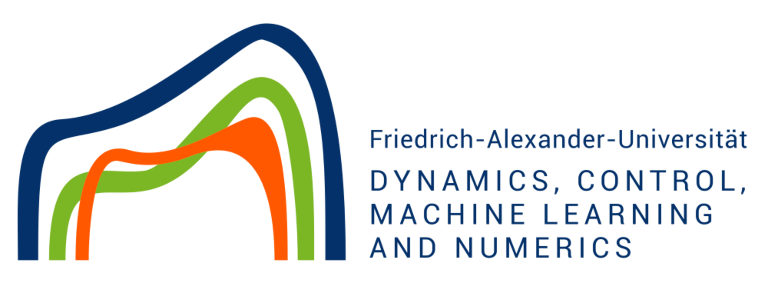### Organizer

###### Website
http://www.dcn.nat.fau.eu

### Speakers

•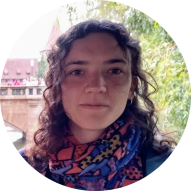Charlotte Rodriguez
•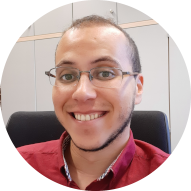Ilias Ftouhi
• Marcos Matabuena
•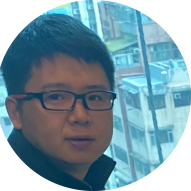Yongcun Song
FAU DCN-AvH | Postdoc